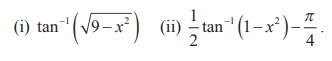Home | | Maths 12th Std | Exercise 4.3: Tangent Function and the Inverse Tangent Function

# Exercise 4.3: Tangent Function and the Inverse Tangent Function

Maths Book back answers and solution for Exercise questions - Mathematics : Inverse Trigonometric Functions: Tangent Function and the Inverse Tangent Function: Exercise Questions with Answer, Solution

EXERCISE 4.3

1.Find the domain of the following functions :2. Find the value of3. Find the value of4. Find the value of